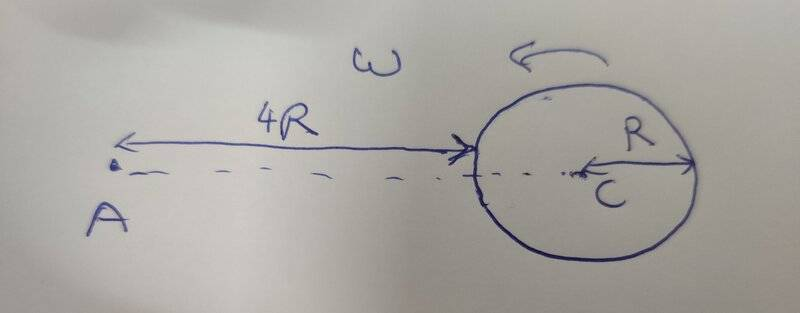# Angular momentum of a disk about an axis parallel to center of mass axis

vcsharp2003
Homework Statement:
A uniform disk of mass M and radius R is rotating at an angular velocity of ##\omega## about it's center C. What is the angular momentum of the disk about an axis passing through A and perpendicular to the plane of disk.
Relevant Equations:
##I_c=\frac {MR^2} {2}##
##\vec L = I \vec {\omega}##
I am using the following formula to solve this problem.
$$L_a= L_c + \text { (angular momentum of a particle at C of mass M)}$$
Because the point C is at rest relative to point A, so the second term in RHS of above equation is zero. Hence, the angular momentum about A is same as angular momentum about it's center of mass C.

$$\therefore L_a = \frac{MR^2}{2} ~ \omega$$.

I am not sure if above conclusion is correct.Homework Helper
Gold Member
It is correct. You can show formally that the angular momentum of an object about an arbitrary point P is the angular momentum about the object's center of mass plus the angular momentum of the center mass about point P. Here, the latter term is zero as you noted.

•vcsharp2003
vcsharp2003
It is correct. You can show formally that the angular momentum of an object about an arbitrary point P is the angular momentum about the object's center of mass plus the angular momentum of the center mass about point P. Here, the latter term is zero as you noted.
Since ##L = I \omega##, can we say that the angular velocity of the disk about A axis is same as the angular velocity about it's C axis i.e. ##\omega_{a} = \omega##?

Homework Helper
Gold Member
Since ##L = I \omega##, can we say that the angular velocity of the disk about A axis is same as the angular velocity about it's C axis i.e. ##\omega_{a} = \omega##?
The angular velocity is the range of change of angle with respect to time. In this case consider angle
##\theta## formed by a reference line, say along the horizontal and the line from C to a point P on the disk that rotates with it. Then you can say that ##\omega_0=\frac{d\theta}{dt}##. Now draw a line from A to P. That line forms angle ##\phi## relative to the horizontal and the angular velocity of P (not of the disk) will be ##\omega=\frac{d\phi}{dt}##. You have to show that ##\omega=\omega_0## for any point P on the disk.

•vcsharp2003
vcsharp2003
The angular velocity is the range of change of angle with respect to time. In this case consider angle
##\theta## formed by a reference line, say along the horizontal and the line from C to a point P on the disk that rotates with it. Then you can say that ##\omega_0=\frac{d\theta}{dt}##. Now draw a line from A to P. That line forms angle ##\phi## relative to the horizontal and the angular velocity of P (not of the disk) will be ##\omega=\frac{d\phi}{dt}##. You have to show that ##\omega=\omega_0## for any point P on the disk.
In general, the angle ##\theta## and ##\phi## will not be equal, so the two angular velocities would have different magnitudes.

•